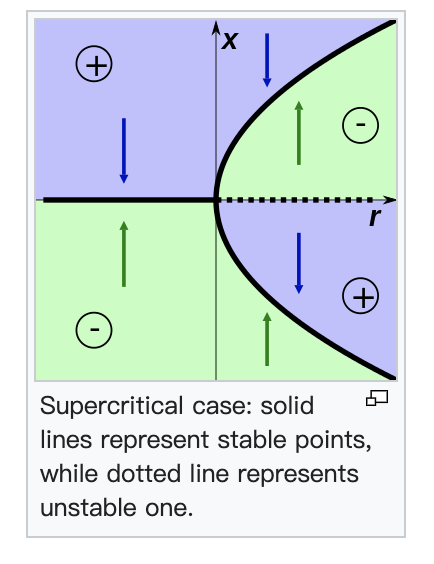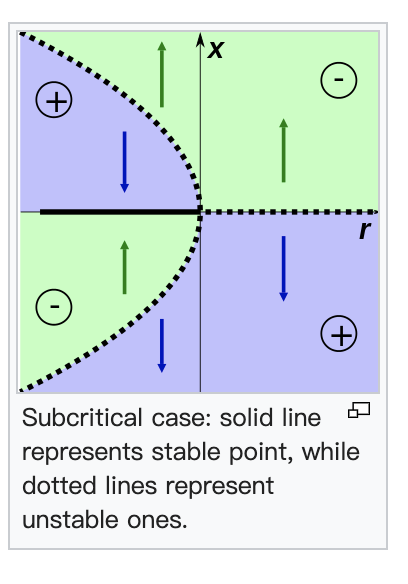# “Bifurcation”

## Saddle-Node bifurcation

### Definition:

In the mathematical area of bifurcation theory a saddle-node bifurcation is a local bifurcation in which two fixed points (or equilibria) of a dynamical system collide and annihilate each other. If the phase space is one-dimensional, one of the equilibrium points is unstable (the saddle), while the other is stable (the node).

### Normal form:

A typical example of a differential equation with a saddle-node bifurcation is: $\frac{d x}{d t}=r+x^{2}$

Here $x$ is the state variable and $r$ is the bifurcation parameter.

• If $r<0$ there are two equilibrium points, a stable equilibrium point at $-\sqrt{-r}$ and an unstable one at $+\sqrt{-r}$.

• At $r=0$ (the bifurcation point) there is exactly one equilibrium point. At this point the fixed point is no longer hyperbolic. In this case the fixed point is called a saddle-node fixed point.

• If $r>0$ there are no equilibrium points

### Example in higher dimensions

An example of a saddle-node bifurcation in two dimensions occurs in the two-dimensional dynamical system:

[\begin{aligned} \frac{d x}{d t} &=\alpha-x^{2} \ \frac{d y}{d t} &=-y \end{aligned}]

## Transcritical bifurcation

### Definition

A transcritical bifurcation is a particular kind of local bifurcation, meaning that it is characterized by an equilibrium having an eigenvalue whose real part passes through zero.

A transcritical bifurcation is one in which a fixed point exists for all values of a parameter and is never destroyed. However, such a fixed point interchanges its stability with another fixed point as the parameter is varied. In other words, both before and after the bifurcation, there is one unstable and one stable fixed point. However, their stability is exchanged when they collide. So the unstable fixed point becomes stable and vice versa.

### Normal Form

The normal form of a transcritical bifurcation is

[\frac{d x}{d t}=r x-x^{2}]

This equation is similar to the logistic equation but in this case we allow $r$ and $x$ to be positive or negative (while in the logistic equation $x$ and $r$ must be non-negative). The two fixed points are at $x=0$ and $x=r$. When the parameter $r$ is negative, the fixed point at $x=0$ is stable and the fixed point $x=r$ is unstable. But for $r>0$, the point at $x=0$ is unstable and the point at $x=r$ is stable. So the bifurcation occurs at $r=0$.

## Pitchfork bifurcation

### Definition

A pitchfork bifurcation is a particular type of local bifurcation where the system transitions from one fixed point to three fixed points. Pitchfork bifurcations, have two types – supercritical and subcritical.

In continuous dynamical systems described by ODEs, i.e. flows—pitchfork bifurcations occur generically in systems with symmetry.

### Supercritical case

The normal form of the supercritical pitchfork bifurcation is

[\frac{d x}{d t}=r x-x^{3}]

For negative values of $r$, there is one stable equilibrium at $x=0$. For $r>0$ there is an unstable equilibrium at $x=0$, and two stable equilibria at $x=\pm \sqrt{r}$.### Subcritical case

The normal form for the subcritical case is

[\frac{d x}{d t}=r x+x^{3}]

In this case, for $r<0$ the equilibrium at $x=0$ is stable, and there are two unstable equilibria at $x=\pm \sqrt {-r}$. For $r>0$ the equilibrium at $x=0$ is unstable.wikipedia

## Hopf bifurcation

### Definition

A Hopf bifurcation is a critical point where a system’s stability switches and a periodic solution arises. More accurately, it is a local bifurcation in which a fixed point of a dynamical system loses stability, as a pair of complex conjugate eigenvalues - of the linearization around the fixed point - crosses the complex plane imaginary axis. Under reasonably generic assumptions about the dynamical system, a small-amplitude limit cycle branches from the fixed point.

### Overview

#### Supercritical and subcritical Hopf bifurcations

The limit cycle is orbitally stable if a specific quantity called the first Lyapunov coefficient is negative,and the bifurcation is supercritical. Otherwise it is unstable and the bifurcation is subcritical. The normal form of a Hopf bifurcation is:

 [\frac{d z}{d t}=z\left((\lambda+i)+b z ^{2}\right)]

where $z$, $b$ are both complex and $\lambda$ is a parameter.

Note : $b=\alpha +i\beta$ , The number $\alpha$ is called the first Lyapunov coefficient.

If $\alpha$ is negative then there is a stable limit cycle for $\lambda > 0$. The bifurcation is then called supercritical. If $\alpha$ is positive then there is an unstable limit cycle for $\lambda < 0$. The bifurcation is called subcritical.## YONG HUANG

Hi, I'm Yong Huang. I've recently graduated from Cornell Tech and obtained my master's degree, I shall start my Ph.D. in Computer Science this fall at UC Irvine. Thank you for visiting my site.